# A Demonstration of Data Mining

REPOST (now in HTML in addition to the original PDF).

This paper demonstrates and explains some of the basic techniques used in data mining. It also serves as an example of some of the kinds of analyses and projects Win Vector LLC engages in.

### John Mount1

A Demonstration of Data Mining

# A Demonstration of Data Mining

## 1  Introduction

A major industry in our time is the collection of large data sets in preparation for the magic of data mining [Loh09,HNP09]. There is extreme excitement about both the possible applications (identifying important customers, identifying medical risks, targeting advertising, designing auctions and so on) and the various methods for data mining and machine learning. To some extent these methods are classic statistics presented in a new bottle. Unfortunately, the concerns, background and language of the modern data-mining practitioner are different than that of the classic statistician- so some demonstration and translation is required. In this writeup we will show how much of the magic of current data mining and machine learning can be explained in terms of statistical regression techniques and show how the statistician’s view is useful in choosing techniques.

Too often data mining is used as a black-box. It is quite possible to clearly use statistics to understand the meaning and mechanisms of data mining.

## 2  The Example Problem

Throughout this writeup we will work on a single idealized example problem. For our problem we will assume we are working with a company that sells items and that this company has recorded its past sales visits. We assume they recorded how well the prospect matched the product offering (we will call this “match factor”), how much of a discount was offered to the prospect (we will call this “discount factor”) and if the prospect became a customer or not (this is our determination of positive or negative outcome). The goal is to use this past record as “training data” and build a model to predict the odds of making a new sale as a function of the match factor and the discount factor. In a perfect world the historic data would look a lot like Figure 1. In Figure 1 each icon represents a past sales-visit, the red diamonds are non-sales and the green disks are successful sales. Each icon is positioned horizontally to correspond to the discount factor used and vertically to correspond to the degree of product match estimated during the prospective customer visit. This data is literally too good to be true in at least three qualities: the past data covers a large range of possibilities, every possible combination has already been tried in an orderly fashion and the good and bad events “are linearly separable.” The job of the modeler would then be to draw the separating line (shown in Figure 1) and label every situation above and to the right of the separating line as good (or positive) and every situation below and to the left as bad (or negative).Figure 1: Ideal Fitting Situation

In reality past data is subject to what prospects were available (so you are unlikely to have good range and an orderly layout of past sales calls) and also heavily affected by past policy. An example policy might be that potential customers with good product match factor may never have been offered a significant discount in the past; so we would have no data from that situation. Finally each outcome is a unique event that depends on a lot more than the two quantities we are recording- so it is too much to hope that the good prospects are simply separable from the bad ones.

Figure 1 is a mere cartoon or caricature of the modeling process, but it represents the initial intuition behind data mining. Again: the flaws in Figure 1 represent the implicit hopes of the data miner. The data miner wishes that the past experiments are laid out in an orderly manner, data covers most of the combinations of possibilities and there is a perfect and simple concept ready to be learned.

Frankly, an experienced data miner would feel incredibly fortunate if the past data looked anything like what is shown in Figure 2.Figure 2: Empirical Data

The green disks (representing good past prospects) and the red diamonds (representing bad past prospects) are intermingled (which is bad). There is some evidence that past policy was to lower the discount offered as the match factor increased (as seen in the diagonal spread of the green disks). Finally we see the red diamonds are also distributed differently than the green disks. This is both good and bad. The good is that the center of mass of the red diamonds differs from the center of mass of the green disks. The bad is that the density of red diamonds does not fall any faster as it passes into the green disks than it falls in any other direction. This indicates there is something important and different (and not measured in our two variables) about at least some of the bad prospects. It is the data miner’s job be aware and to press on.

### 2.1  The Trendy Now

In truth data miners often rush where classical statisticians fear to tread. Right now the temptation is to immediately select from any number of “red hot” techniques, methods or software packages. My short list of super-star method buzzwords includes:

Based on some of the above referenced writing and analysis I would first pick “logistic regression” as I am confident that, when used properly, it is just about as powerful as any of the modern data mining techniques (despite its somewhat less than trendy status). Using logistic regression I immediately get just about as close to a separating line as this data set will support: Figure 3.Figure 3: Linear Separator

The separating line actually encodes a simple rule of the form: “if 2.2*DiscountFactor + 3.1*MatchFactor ≥ 1 then we have a good chance of a sale.” This is classic black-box data mining magic. The purpose of this writeup is to look deeper how to actually derive and understand something like this.

## 3  Explanation

What is really going on? Why is our magic formula at all sensible advice, why did this work at all and what motivates the analysis? It turns out regression (be it linear regression or logistic regression) works in this case because it somewhat imitates the methodology of linear discriminant analysis (described in: [Fis36]). In fact in many cases it would be a better idea to perform a linear discriminant analysis or perform an analysis of variance than to immediately appeal to a complicated method. I will first step through the process of linear discriminant analysis and then relate it to our logistic regression. Stepping through understandable stages lets us see where we were lucky in modeling and what limits and opportunities for improvement we have.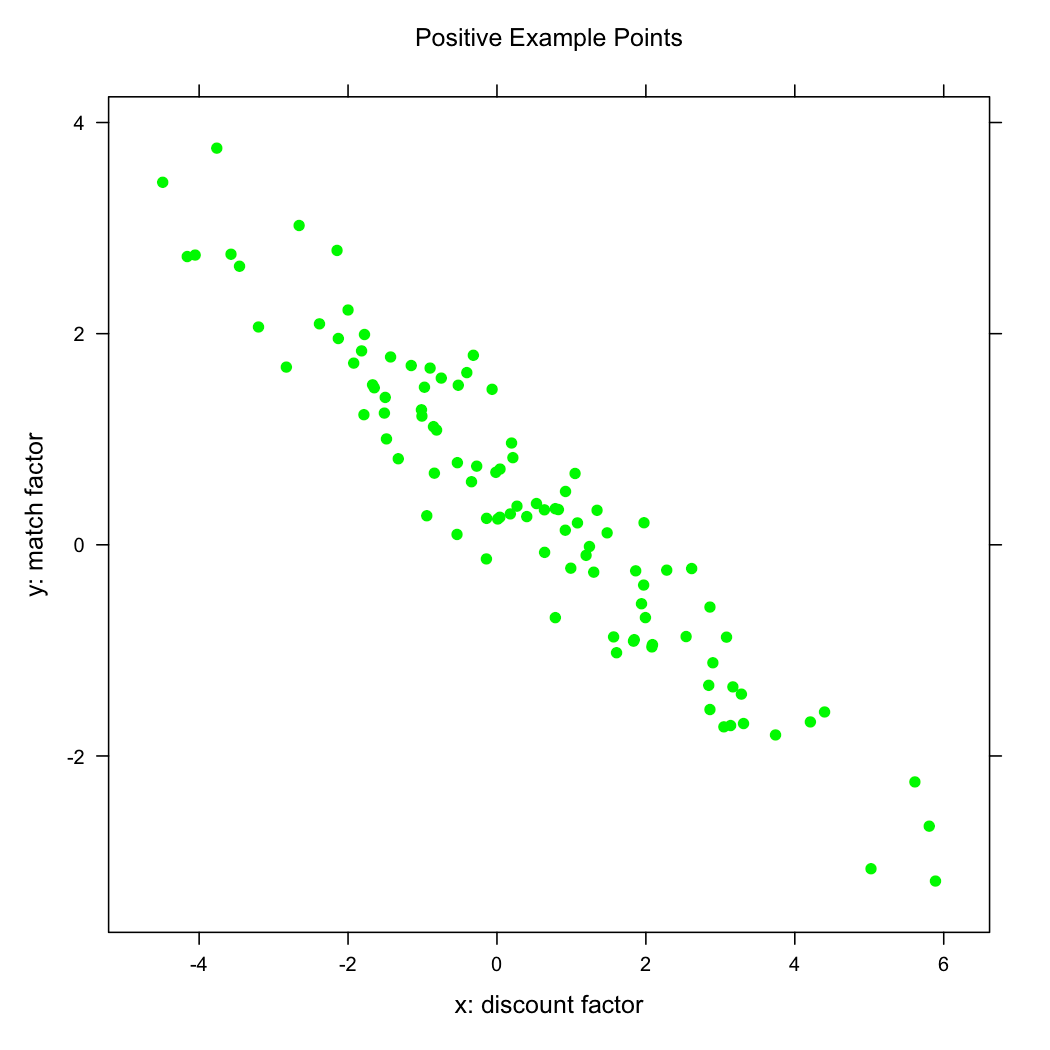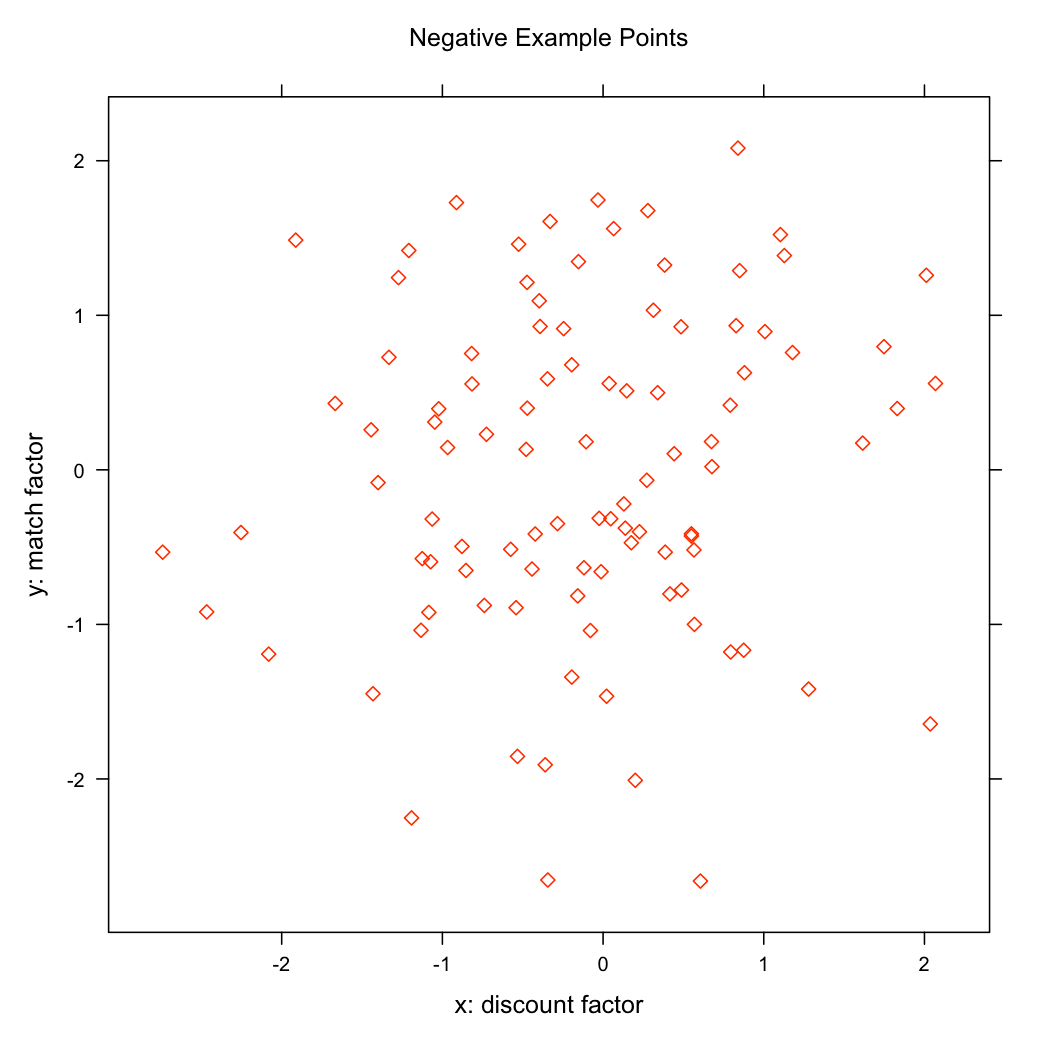Figure 4: Separate Plots

Our data initially looks very messy (the good and bad group are fairly mixed together). But if we examine out data in separate groups we can see we are actually incredibly lucky in that the data is easy to describe. As we can see in Figure 4: the data, when separated by outcome (plotting only all of the good green disks or only all of the bad red diamonds), is grouped in simple blobs without bends, intrusions or other odd (and more work to model) configurations.

We can plot the idealizations of these data distributions (or densities) as “contour maps” (as if we are looking down on the elevations of a mountain on a map) which gives us Figure 5.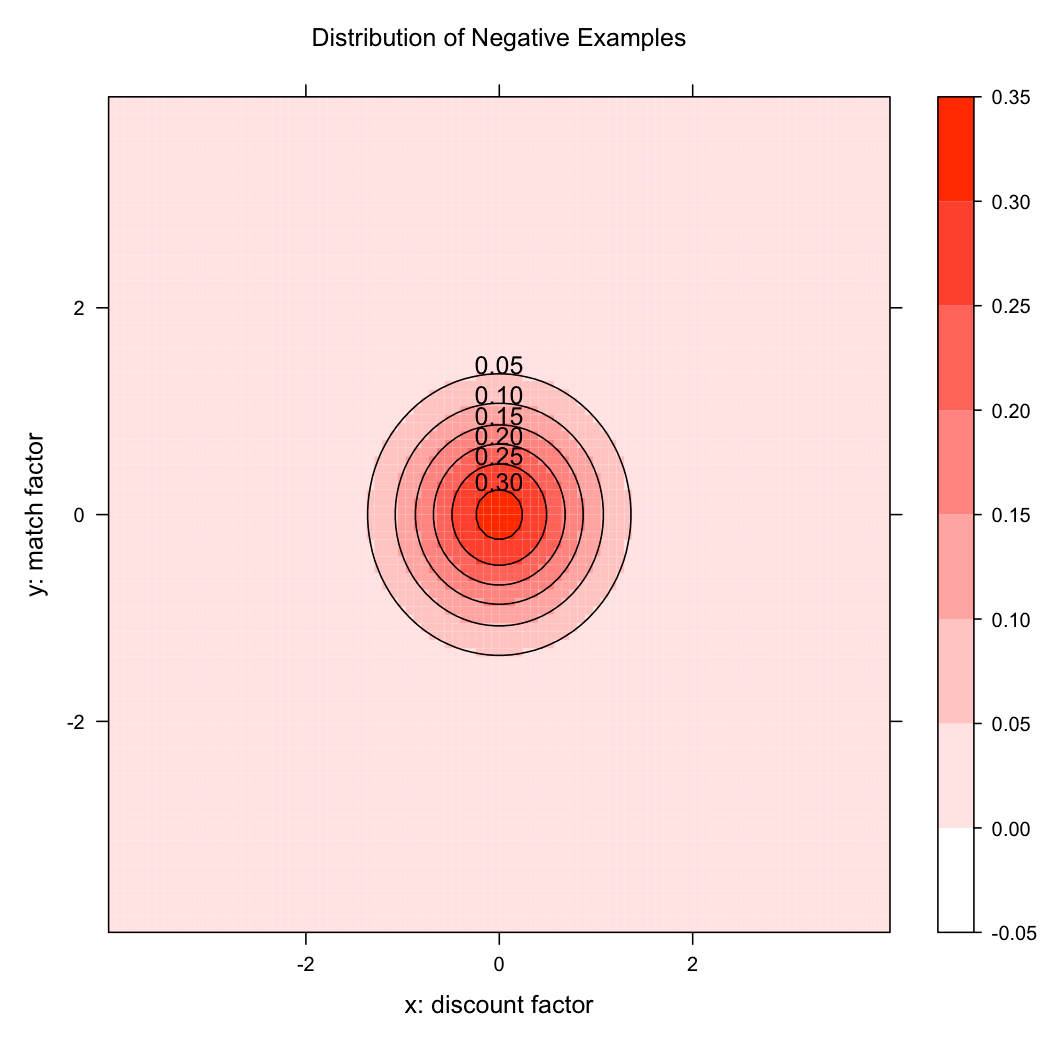Figure 5: Separate Distributions

### 3.1  Full Bayes Model

From Figure 5 we can see while our data is not separable there are significant differences between the groups. The difference in the groups is more obvious if we plot the difference of the densities on the same graph as in Figure 6. Here we are visualizing the distribution of positive examples as a connected pair of peaks (colored green) and the distribution of negative examples a deep valley (colored red) located just below and to the left of the peaks.Figure 6: Difference in Density

This difference graph is demonstrating how both of the densities or distributions (positive and negative) reach into different regions of the plane. The white areas are where the difference in densities is very small which includes the areas in the corners (where there is little of either distribution) and the area between the blobs (where there is a lot of mass from both distributions competing). This view is a bit closer to what a statistician wants to see- how the distributions of successes and failures different (this is a step to take before even guessing at or looking for causes and explanations).

Figure 6 is already an actionable model- we can predict the odds a new prospect will buy or not at a given discount by looking where they fall on Figure 6 and checking if they fall in a region on strong red or strong green color. We can also recommend a discount for a given potential customer by drawing a line at the height determined by their degree of match and tracing from left to right until we first hit a strong green region. We could hand out a simplified Figure 7 as a sales rulebook.Figure 7: Full Bayes Model

This model is a full Bayes model (but not a Naive Bayes model, which is oddly more famous and which we will cover later). The steps we took were: first we summarized or idealized our known data into two Gaussian blobs (as depicted in Figure 5). Once we had estimated the centers, widths and orientations of these blobs we could then: for any new point say how likely the point is under the modeled distribution of sales and how likely the point is under the modeled distribution of non-sales. Mathematically we claim we can estimate P(x,y |sale)2 and P(x,y | non-sale) (where x is our discount factor and y is our matching factor).3 Neither of these are what we are actually interested in (we want: P(sale | x,y)4). We can, however, use these values to calculate what we want to know. Bayes’ law is a law of probability that says if we know P(sale | x,y), P(non-sale | x,y), P(sale) and P(non-sale)5 then: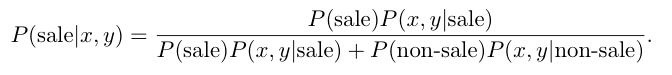Figure 7 depicts a central hourglass shaped region (colored green) that represents the region of x, y values where P(sale |x,y) is estimated to be at least 0.5 and the remaining (darker red region) are the situations predicted to be less favorable. Here we are using priors of P(sale) = P(non-sale) = 0.5, for different priors and thresholds we would get different graphs.

Even at this early stage in the analysis we have already accidentally introduced what we call “an inductive bias.” By modeling both distributions as Gaussians we have guaranteed that our acceptance region will be an hourglass figure (as we saw in Figure 7). One undesirable consequence of the modeling technique is the prediction sales become unlikely when both match factor and discount factor are very large. This is somewhat a consequence of our modeling technique (though the fact that the negative data does not fall quickly as it passes into the green region also added to this). This un-realistic (or “not physically plausible”) prediction is called an artifact (of the technique and of the data) and it is the statistician’s job to see this, confirm they don’t want it and eliminate it (by deliberately introducing a “useful modeling bias”).

### 3.2  Linear Discriminant

To get around the bad predictions of our model in the upper-right quadrant we “apply domain knowledge” and introduce a useful modeling bias as follows. Let us insist that our model be monotone: that if moving some direction is good than moving further in the same direction is better. In fact let’s insist that our model be a half-plane (instead of two parabolas). We want a nice straight separating cut, which brings us to linear discriminant analysis. We have enough information to apply Fisher linear discriminant technique and find a separator that maximizes the variance of data across categories while minimizing the variance of data within one category and within the other category. This is called the linear discriminant and it is shown in Figure 8.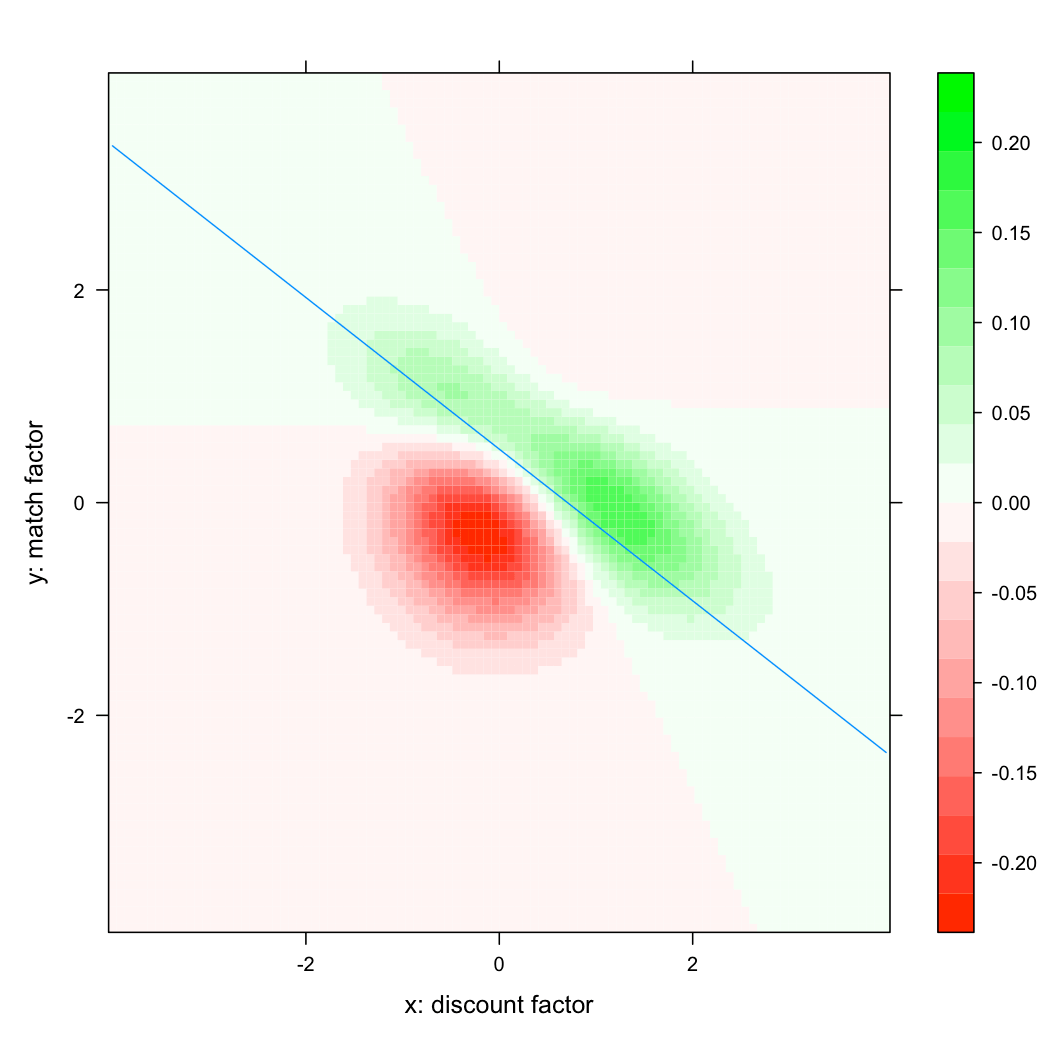Figure 8: Linear Discriminant

The blue line is the linear discriminant (similar to the logistic regression line depicted earlier on the data-slide). Everything above or to the right of the blue line is considered good and everything below or to the left of the blue line is considered bad. Notice that this advice while not quite as accurate as the Bayes Model near the boundary between the two distributions is much more sensible about the upper right corner of the graph.

To evaluate a separator we collapse all variation parallel to the separating cut (as shown in Figure 9). We then see that each distribution becomes a small interval or streak. A separator is good if these resulting streaks are both short (the collapse packs the blobs) and the two centers of the streaks are far apart (and on opposite size of the separator). In Figure 9 the streaks are fairly short and despite some overlap we do have some usable separation between the two centers.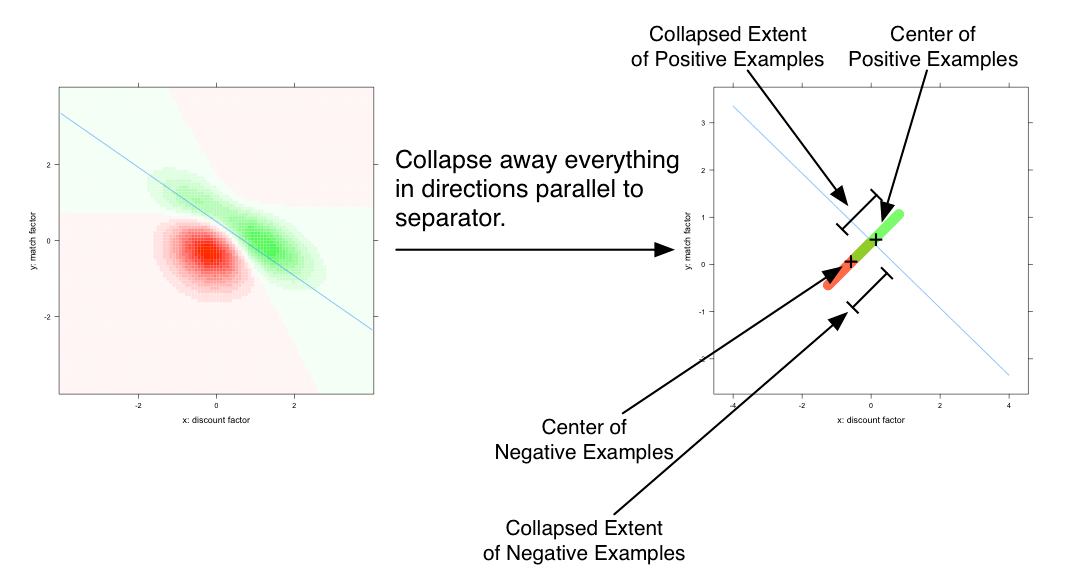Figure 9: Evaluating Quality of Separating Cut

To make the above precise we switch to mathematical notation. For the i-th positive training example form the vector v+,i and the matrix S+,i where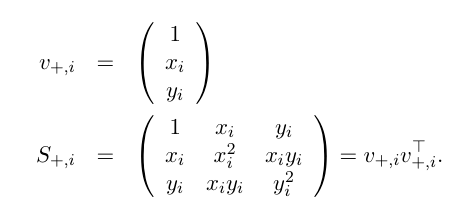where xi and yi are the known x and y coordinates for this particular past experience. Define v−,i, S−,i similarly for all negative examples. In this notation we have for a direction γ: the distance along the γ direction between the center of positive examples and center of negative examples is: γT ( ∑i v+,i / n+ − ∑i v−,i / n) (where n+ is the number of positive examples and n is the number of negative examples). We would like this quantity to be large. The degree of spread or variance of the positive examples along the γ direction is γT (∑i S+,i / n+) γ. The degree of spread or variance of the negative examples along the γ direction is γT (∑i S−,i / n) γ. We would like the last two quantities to be small. The linear discriminant is picked to maximize: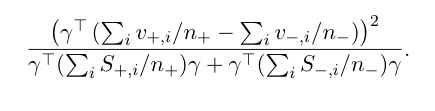It is a fairly standard observation (involving the Rayleigh quotient) that this form is maximized when:As we have said, the linear discriminant is very similar to what is returned by a regression or logistic regression. In fact in our diagrams the regression lines are almost identical to the linear discriminant. A large part of why regression can be usefully applied in classification comes from its close relationship to the linear discriminant.

### 3.3  Linear Regression

Linear regression is designed to model continuous functions subject to independent normal errors in observation. Linear regression is incredibly powerful at characterizing and elimination correlations between the input variables of a model. While function fitting is different than classification (our example problem) linear regression is so useful whenever there is any suspected correlation (which is almost always the case) that it is an appropriate tool. In our example in the positive examples (those that led to sales) there is clearly a historical dependence between the degree of estimated match and amount of discount offered. Likely this dependence is from past prospects being subject to a (rational) policy of “the worse the match the higher the offered discount” (instead of being arranged in a perfect grid-like experiment as in our first diagram: Figure 1). If this dependence is not dealt with we would under-estimate the value of discount because we would think that discounted customers are not signing up at a higher rate (when these prospects are in fact clearly motivated by discount, once you control for the fact that many of the deeply discounted prospects had a much worse degree of match than average).

For analysis of categorical data linear regression is closely linked to ANOVA (analysis of variance).[Agr02] Recall that variance was a major consideration with the linear discriminant analysis, so we should by now be on familiar ground.

In our notation the standard least-squares regression solution is:where y+,i = 1 for all i and y−,i = −1 for all i.

If we have the same number of positive and negative examples (i.e. n+ = n) then Equation 1 and Equation 2 are identical and we have β = γ. So in this special case the linear discriminant equals the least square linear regression solution. We can even ask how the solutions change if the relative proportions of positive and negative training data changes. The linear discriminant is carefully designed not to move, but the regression solution will tilt to be an angle that is more compatible with the larger of the example classes and shift to cut less into that class. The linear regression solution can be fixed (by re-weighting the data) to also be insensitive to the relative proportions of positive and negative examples but does not behave that way “fresh out of the box.”

### 3.4  Logistic Regression

While linear regression is designed to pick a function that minimizes the sum of square errors logistic regression is designed to pick a separator that maximizes something called the plausibility of the data. In our case since the data is so well behaved the logistic regression line is essentially the same as the linear regression line. It is in fact an important property of logistic regression that there is always a re-weighting (or choice of re-emphasis) of the data that causes some linear regression to pick the same separator as the logistic regression. Because linear and logistic regression are only identical in specific circumstances it is the job of the statistician to know which of the two is more appropriate for a given data set and given intended use of the resulting model.

## 4  Other Methods and Techniques

### 4.1  Kernelized Regression

One way to greatly expand the power of modeling methods is a trick called kernel methods. Roughly kernel methods are those methods that increase the power of machine learning by moving from a simple problem space (like ours in variables x and y) to a richer problem space that may be easier to work in. A lot of ink is spilled about how efficient the kernel methods are (they work in time proportional to the size of the simple space, not the complex one) but this is not their essential feature. The essential feature is the expanded explanation power and this is so important that even the trivial kernel methods (such as directly adjoining additional combinations of variables) pick up most of the power of the method. Kernel methods are also overly associated with Support Vector Machines- but are just as useful when added to Naive Bayes, linear regression or logistic regression.

For instance: Figure 10 shows a bow-tie like acceptance region found by using linear regression over the variables x, y, x2, y2 and x y (instead of just x and y). Note how this result is similar to the full Bayes model (but comes from a different feature set and fitting technique).Figure 10: Kernelized Regression

### 4.2  Naive Bayes Model

We briefly return to the Bayes model to discuss a more common alternative called “Naive Bayes.” A Naive Bayes model is like a full Bayes model except an additional modeling simplification is introduced in assuming that P(x,y|sale) = P(x|sale)P(y|sale) and P(x,y|non-sale) = P(x|non-sale)P(y|non-sale). That is we are assuming that the distributions of the x and y measurements are essentially independent (once we know which outcome happened). This assumption is the opposite of what we do with regression in that we ignore dependencies in the data (instead of modeling and eliminating the dependencies). However, Naive Bayes methods are quite powerful and very appropriate in sparse-data situations (such as text classification). The “naive” assumption that the input variables are independent greatly reduces the amount of data that needs to be tracked (it is much less work to track values of variables instead of simultaneous values of pairs of variables). The curved separator from this Naive Bayes model is illustrated in Figure 11.Figure 11: Naive Bayes Model

The Naive Bayes version of the advice or policy chart is always going to be an axis-aligned parabola as in Figure 12. Notice how both the linear discriminant and the Naive Bayes model make mistakes (places some colors on the wrong side of the curve)- but they are simple, reliable models that have the desirable property of having connected prediction regions.Figure 12: Naive Bayes Decision

### 4.3  More Exotic Methods

Many of the hot buzzword machine learning and data mining methods we listed earlier are essentially different techniques of fitting a linear separator over data. These methods seem very different but they all form a family once you realize many of the details of the methods are determined by:

• Choice of Loss Function

This is what notion of “goodness of fit” is being used. It can be normalized mean-variance (linear discriminants), un-normalized variance (linear regression), plausibility (logistic regression), L1 distance (support vector machines, quantile regression), entropy (maximum entropy), probability mass and so on.

• Choice of Optimization Technique

For a given loss function we can optimize in many ways (though most authors make the mistake of binding their current favorite optimization method deep into their specification of technique): EM, steepest descent, conjugate gradient, quasi-Newton, linear programming and quadratic programming to name a few.

• Choice of Regularization Method

Regularization is the idea of forcing the model to not pick extreme values of parameters to over-fit irrelevant artifacts in training data. Methods include MDL, controlling energy/entropy, Lagrange smoothing, shrinkage, bagging and early termination of optimization. Non-explicit treatment of regularization is one reason many methods completely specify their optimization procedure (to get some accidental regularization).

• Choice of Features/Kernelization

The richness of the feature set the method is applied to is the single largest determinant of model quality.

• Pre-transformation Tricks

Some statistical methods are improved by pre-transforming the outcome data to look more normal or be more homoscedastic.6

If you think along a few axes like these (instead of evaluating them by their name and lineage) you tend to see different data mining methods more as embodying different trade-offs than as being unique incompatible disciplines.

## 5  Conclusion

Our goal for this writeup was to fully demonstrate a data mining method and then survey some important data mining and machine learning techniques. Many of the important considerations are “too obvious” to be discussed by statisticians and “too statistical” to be comfortably expressed in terms popular with data miners. The theory and considerations from statistics when combined with the experience and optimism of data-mining/machine-learning truly make possible achieving the important goal of “learning from data.”

This expository writeup is also meant to serve as an example of the
types of research, analysis, software and training supplied by
Win-Vector LLC http://www.win-vector.com . Win-Vector LLC
prides itself in depth of research and specializes in identifying,
documenting and implementing the “simplest technique that can
possibly work” (which is often the most understandable, maintainable,
robust and reliable). Win-Vector LLC specializes in research but
has significant experience in delivering full solutions (including
software solutions and integration with existing databases).

## References

[Agr02]
Alan Agresti, Categorical data analysis (wiley series in probability and
statistics)
, Wiley-Interscience, July 2002.

[BF97]
Leo Breiman and Jerome H Friedman, Predicting multivariate responses in
multiple linear regression
, Journal of the Royal Statistical Society, Series
B (Methodological) 59 (1997), no. 1, 3-54.

[BNJ03]
David M Blei, Andrew Y Ng, and Michael I Jordan, Latent dirichlet
allocation
, Journal of Machine Learning Research 3 (2003),
993-1022.

[Bre00]
Leo Breiman, Special invited paper. additive logistic regression: A
statistical view of boosting: Discussion
, Ann. Statist. 28 (2000),
no. 2, 374-377.

[BRS08]
Richard Beigel, Nick Reingold, and Daniel A Spielman, The perceptron
strikes back
, 6.

[CST00]
Nello Cristianini and John Shawe-Taylor, An introduction to support
vector machines and other kernel-based learning methods
, 1 ed., Cambridge
University Press, March 2000.

[DKM05]
Sanjoy Dasgupta, Adam Tauman Kalai, and Claire Monteleoni, Analysis of
perceptron-based active learning
, CSAIL Tech. Report (2005), 16.

[DS06]
Miroslav Dudik and Robert E Schapire, Maximum entropy distribution
estimation with generalized regularization
, COLT (2006), 15.

[Fis36]
Ronald A Fisher, The use of multiple measurements in taxonomic problems,
Annals of Eugenics 7 (1936), 179-188.

[FISS03]
Yoav Freund, Raj Iyer, Robert E Schapire, and Yoram Singer, An efficient
boosting algorithm for combining preferences
, Journal of Machine Learning
Research 4 (2003), 933-969.

[FPP07]
David Freedman, Robert Pisani, and Roger Purves, Statistics 4th edition,
W. W. Norton and Company, 2007.

[Gru05]
Peter D Grunwald, Maximum entropy and the glasses you are looking
through
.

[HNP09]
Alon Halevy, Peter Norvig, and Fernando Pereira, The unreasonable
effectiveness of data
, IEEE Intellegent Systems (2009).

[KM03]
Dan Klein and Christopher D Manning, Maxent models, conditional
estimation, and optimization
.

[Koe05]
Roger Koenker, Quantile regression, Cambridge University Press, May
2005.

[Lew98]
David D Lewis, Naive (bayes) at forty: The independence assumption in
information retrieval
.

[Loh09]
Steve Lohr, For today’s graduate, just one word: Statistics,
http://www.nytimes.com/2009/08/06/technology/06stats.html, August 2009.

[Sar08]
Deepayan Sarkar, Lattice: Multivariate data visualization with R,
Springer, New York, 2008, ISBN 978-0-387-75968-5.

[SC89]
Hal Stern and Thomas M Cover, Maximum entropy and the lottery, Journal
of the American Statistical Association 84 (1989), no. 408,
980-985.

[Sch01]
Robert E Schapire, The boosting approach to machine learning an
overview
, 23.

[STC04]
John Shawe-Taylor and Nello Cristianini, Kernel methods for pattern
analysis
, Cambridge University Press, June 2004.

APPENDIX

## A  Graphs

The majority of the graphs in this writeup were produced using “R”
http://www.r-project.org/ and Deepayan Sarkar’s Lattice
package[Sar08].

### Footnotes:

2Read P(A | B) as: “the probability of A will
happen given we know B is true.”

3Technically we are working with densities, not
probabilities, but we will use probability notation for its
intuition.

4P(sale | x,y) is the probability of
making a sale as a function of what we know about the prospective
customer and our offer. Whereas P(x,y|sale) was just how likely it is
to see a prospect with the given x and y values, conditioned on knowing we made
a sale to this prospect.

5 P(sale) and
P(non-sale) are just the “prior odds” of sales or what
our estimate of our chances of success are before we look at any
facts about a particular customer. We can use our historical
overall success and failure rates as estimates of these quantities.

6A situation is homoscedastic if the errors are independent of where we are in the parameter space (our x,y or match factor and discount factor). This property is very important for meaningful fitting/modeling and interpreting significance of fits.

File translated from
TEX
by
TTH
,
version 3.85.
On 29 Aug 2009, 11:43.### jmount

Data Scientist and trainer at Win Vector LLC. One of the authors of Practical Data Science with R.

### 2 replies ›

1.jmount says:

One more graph.

Here we again show the linear discriminant line (solid) we talked about throughout the paper and a new line (dashed) that represents a “best probability” fit.

The new dashed separating line is a cut that maximizes the probability of getting an example point correct (points drawn with odds proportional to the odds described by our fit distributions). This alternate fit is gotten by switching to a “mass based metric” (or a L0 metric) and is similar in spirit to the ideas in Quantile Regression and Support Vector Machines (which use a L1 metric instead of a L2 metric or the more common plausibility measures).

You can see how the new dashed fit line is more compatible with the shape of the lower red region (the negative examples) than the original solid fit line. If you have a good idea what you want you can actually choose a fit line the respects one shape more or the other (depending on what is important in your application).

There will probably be Win-Vector article at some time on the methods to find this fit.

2.Mei Marker says:

Hi John,

Nice article and educational for me!

My 2 cents: I think the full Bayes Model and Kernelized regression result actually makes more sense than “monotone assumption”. The reason is that when the match factor is high, a high discount factor may not be good because when people find exactly what they are looking for, and you sell it too cheap, it may actually turn people away. So upper right corner labeled red actually could be the right thing to do. On top of that, I also favor the underlying assumptions/theories behind full Bayes and Kernel methods.

Regards,
Mei